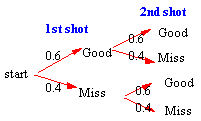### CFA Practice Question

There are 985 practice questions for this topic.

### CFA Practice Question

Joan has a 60% probability of making a free-throw. If she shoots two free-throws, what is the probability she misses both of them?

A. 48%
B. 16%
C. 60%

Drawing a tree diagram, the event Miss AND Miss is represented by the bottom branch. The probability of traveling this branch is (0.4)(0.4) = 0.16 = 16%. Thus, P(Joan misses both free-throws) = 16%.User Comment
tenny45 or let A = make first B= make second
Then P(A and B) = 0.6*0.6 = 0.36
P (A OR B) = 0.6+0.6-0.36 = 0.84
P (NOT A, NOT B) = 1 - 0.84 = 16%

Longer but no graphs.
bobert Or you can simply realize the events are independent of each other which translates to P(AA) = P(A) * P(A) or in this case P(not AA) = P(not A) * P(not A)= P(not AA) = (.4)(.4) = .16

Note: I used P(AA) because there is only 1 event, also indicating no dependence.
Poorvi I did it this way. If p(a) is that she makes the freethrow, then p(a) =0.6 P(a compl) = 1-0.6=0.4
To get two p(a compl) twice, 0.4*0.4=0.16
AUAU y not 1 - 0.60 x 0.60 = 0.64
Zawerthal AUAU, that would be the probability of making both free throws
gyee2012 ^ I like tenny45's logic for an easy check
The or statement pf P(A or B) = .84
Therefore the opposite would equal .16

The faster route is to realize this is independent so the calcuations are straight forward and easy. 0.40^2 = 0.16